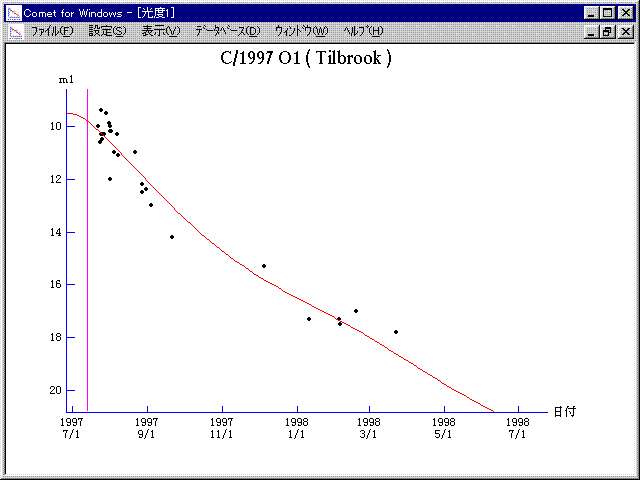# \$B%F%#%k%V%k%C%/WB@1(B

C/1997 O1 ( Tilbrook )###\$B%W%m%U%#!<%k(B

 \$BH/8+F|(B 1997\$BG/(B7\$B7n(B22\$BF|(B \$BH/8+8wEY(B 10\$BEy(B \$BH/8+ J. Tillbrook (Clare, S. Australia)

###\$B50F;MWAG(B

```   The following improved orbital elements by Kenji Muraoka, are
from 111 observations 1997 July 23 to 1998 Mar. 23, perturbations
by 9 Planets, Moon and 5 minor planets were taken into account.
The mean residual is +/- 0.67 arc seconds.

Epoch  =  1997 July 11.0  TT       JDT = 2450640.5
T  =  1997 July 13.41925       +/- 0.00021 (m.e.) TT
Peri. =  336.18714                +/- 0.00018
Node  =  231.24573                +/- 0.00013   (2000.0)
Incl. =  115.80202                +/- 0.00019
q  =    1.3718028              +/- 0.0000039 AU
e  =    0.9842429              +/- 0.0000080
1/a  =   +0.0114864              +/- 0.0000058 1/AU
( P  =    812 years )
```

###\$B@1?^(B1997\$BG/(B 5\$B7n(B 2\$BF|!A(B1997\$BG/(B 9\$B7n(B19\$BF|(B1997\$BG/(B 8\$B7n(B10\$BF|!A(B1998\$BG/(B12\$B7n(B23\$BF|(B

###\$B8wEYJQ2=(B

```        m1 = 7.4 + 5 log\$B&\$(B + 16.0 log r
```##### \$B50F;MWAG\$OB<2,7r<#;a\$N7W;;\$K\$h\$k\$b\$N\$G\$9!#(B \$B@1?^\$O(B StellaNavigator Ver.2.0 for Windows (\$B%"%9%H%m%"!<%D(B \$BJTCx(B / \$B%"%9%-!<=PHG6I4)(B) \$B\$G:n@.\$7\$?\$b\$N\$G\$9!#(B \$B8wEY%0%i%U\$O(BComet for Windows\$B\$G:n@.\$7\$?\$b\$N\$G\$9!#(B